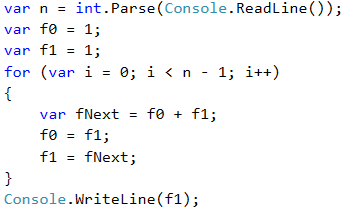# Problem: Fibonacci Numbers

Fibonacci's numbers in mathematics form a sequence that looks like this: 1, 1, 2, 3, 5, 8, 13, 21, 34, ….

The formula to form the Fibonacci sequence is:

F0 = 1
F1 = 1
Fn = Fn-1 + Fn-2


#### Sample Input and Output

Input (n) Output Comment
10 89 F(11) = F(9) + F(8)
5 8 F(5) = F(4) + F(3)
20 10946 F(20) = F(19) + F(18)
0 1 By definition
1 1 By definition

Enter an integer number n and calculate the n-number of Fibonacci.

## Video: Fibonacci Numbers

Watch this video lesson to learn how to calculate the Fibonacci numbers: https://youtu.be/1ZR0ZBFzB3c.

## Hints and Guidelines

An idea to solve the problem:

• We create a variable n to which we assign an integer value from the console input.
• We create the variables f0 and f1 to which we assign a value of 1, since the sequence starts.
• We create a for loop with condition the current value i < n - 1.
• In the body of the loop:
• We create a temporary variable fNext, to which we assign the next number in the Fibonacci sequence.
• To f0 we assign the current value of f1.
• To f1 we assign the value of the temporary variable fNext.
• Out of the loop we print the n-th number of Fibonacci.

Example implementation: